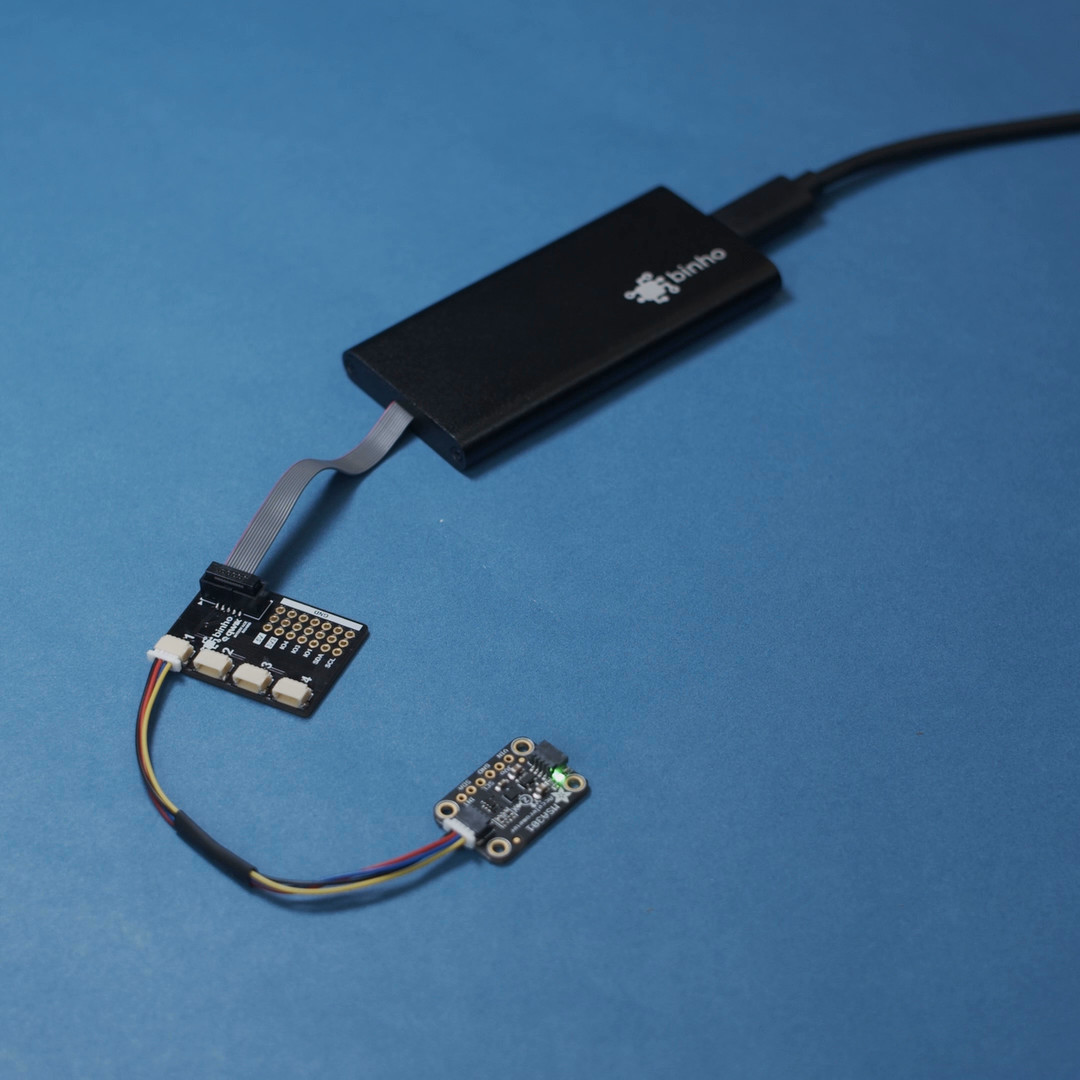The best tools to make your project dreams come true

﻿

# Low-Cost Data Acquisition (DAQ) with Arduino and Binho for ML

## By ShawnHymel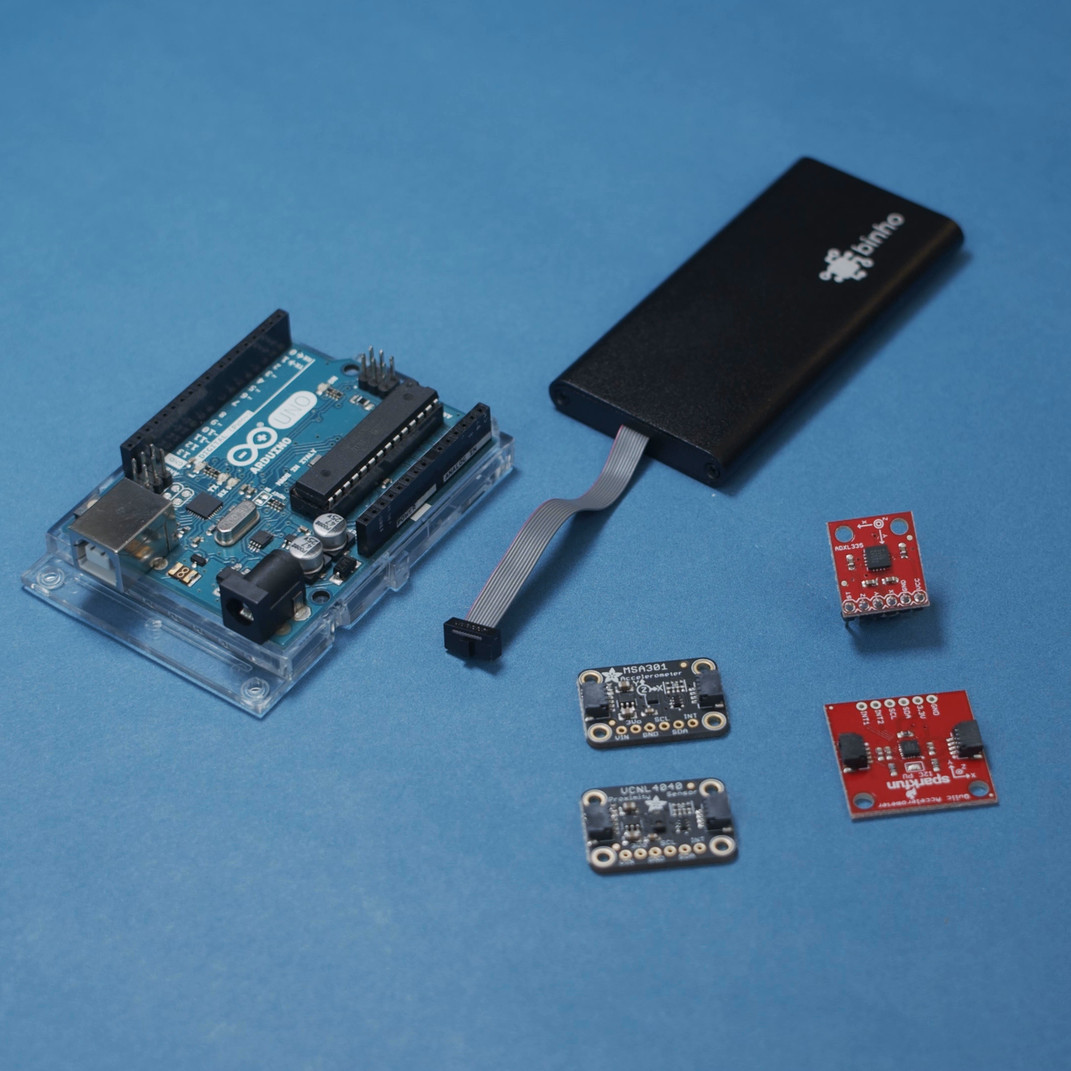Data Acquisition (DAQ) is the process of collecting information from one or more sensors for processing on a computer. Most DAQ devices act as a converter to read from one or more sensors and convert the reading to a raw measurement value. Many DAQ devices can be scripted to read at various intervals, at particular data rates, etc.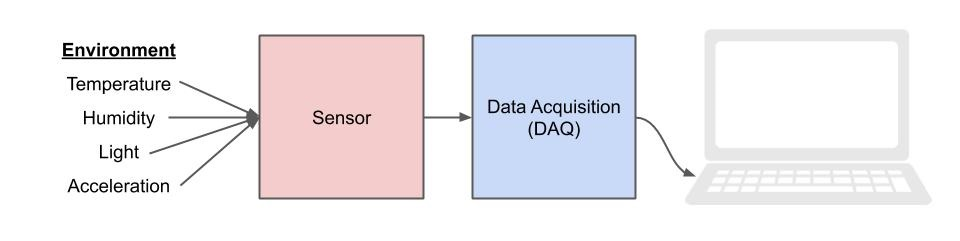Many professional DAQ devices are easily a few hundred to thousands of dollars. If you only need to collect simple data, like from an accelerometer or temperature sensor, we can create our own DAQ device from an Arduino. Additionally, we can step up to the professional Binho Nova host adapter to act as a DAQ as well.

With both of these devices, we can rely on community-written libraries to avoid having to write driver code to communicate with the sensors, which saves us a good amount of time (and staring at datasheets). While we do need to write some code to read from the sensors and DAQ devices, it can prove to be a more economical solution than relying on a professional DAQ.

Collecting sensor data can be extremely important to many machine learning tasks, as you will often need to collect hundreds or thousands of samples. Scripting the collection of these can make the collection process much easier.

You can see how to work with the Arduino and Binho Nova to read from a sensor in video form here:

Arduino DAQ

Arduino has made writing firmware for microcontrollers extremely easy and accessible for many people. While the abstraction layers might be less efficient as writing strict C tailored for your target process, it can make creating a DAQ quick and (relatively) painless. You have access to several different sensor interfaces, including analog-to-digital converters (ADC), I2C, and SPI. Additionally, community-written libraries mean you can spend less time writing low-level firmware drivers.

To start, we’ll write a quick Arduino sketch that reads from an MSA301 accelerometer and prints out the X, Y, and Z acceleration measurements over Serial whenever an ‘r’ character is received. This lets our computer control the sampling rate. You’ll want to note the sampling rate of the accelerometer, so there is a little bit of datasheet reading that’s needed. If we’re using the Adafruit library, the sensor updates its internal values at a rate of 500 Hz by default (which can be changed up to 1000 Hz). So, you’ll need the computer to sample no faster than 500 Hz.

Connect the MSA301 accelerometer board to the Arduino via I2C port:

Copy Code
`Arduino --> MSA301   5V   -->  VIN  GND   -->  GND  SCL   -->  SCL  SDA   -->  SDA`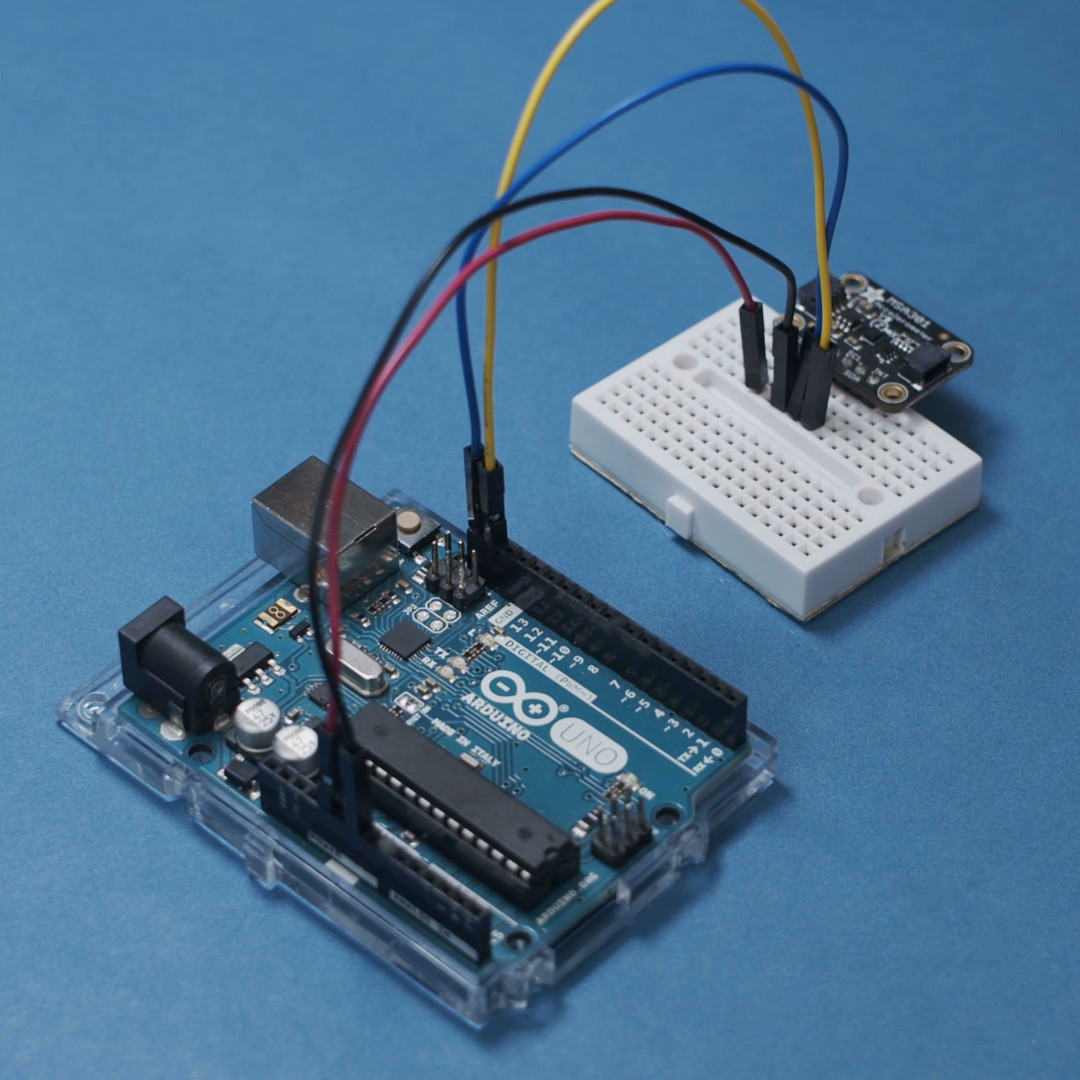In a new Arduino sketch, install the Adafruit MSA301 library, the Adafruit BusIO library, and the Adafruit unified sensor library (as per this tutorial). Enter the following code:

Copy Code
`#include <Wire.h>#include <Adafruit_MSA301.h>#include <Adafruit_Sensor.h>Adafruit_MSA301 msa;void setup() {  // Open serial port  Serial.begin(250000);  // Initialize MSA301  if (!msa.begin()) {    Serial.println("Error: Could not find MSA301 chip");    while (1);  }}void loop() {  char c;  // Wait until we get an 'r' on the serial line  if (Serial.available()) {    c = Serial.read();    if (c == 'r') {            // Get new MSA reading      msa.read();          // Print out results      Serial.print(msa.x_g);      Serial.print('\t');      Serial.print(msa.y_g);      Serial.print('\t');      Serial.println(msa.z_g);    }  }}`

Upload that to your Arduino. I’m using an Arduino UNO in this example, but you’re welcome to use almost any Arduino board (assuming you have an I2C port). Open the Serial monitor. At the top, enter an ‘r’ character and press “Send.” You should see 3 values printed out, corresponding to X, Y, and Z acceleration measurements from the MSA301. Note that the units are g-force.

Read from Arduino DAQ with Python

Since we’re doing this for use in machine learning (ML), I’m going to show the example in Jupyter Notebook. However, you’re welcome to use regular Python as well.

First, we need to install the pyserial package. In a new cell, enter:

Copy Code
`!python -m pip install pyserial`

When that’s done, import a few packages in the next cell:

Copy Code
`import serialimport numpy as npimport matplotlib.pyplot as pltimport time`

We then need to set some parameters. You’ll want to change the serial port to match your Arduino (which you can find in the Arduino IDE):

Copy Code
`# Settingsserial_port = 'COM19'baud_rate = 250000label_name = 'circle'num_class_samples = 10   # Number of samples to collect for this label categorytime_per_sample = 2.0    # Secondsaccel_sample_rate = 100  # Times per second (Hz) to read from acceleromaternum_accel_samples = int(time_per_sample * accel_sample_rate)`

As a test, we’ll want to open the serial port and attempt to read from the Arduino.

Copy Code
`# Test Serial port (open, wait for Arduino to reset, read line, close)ser = serial.Serial(serial_port, baud_rate, timeout=1)time.sleep(2)ser.write('r'.encode('ascii'))line = ser.readline()ser.close()print(line)`

Seeing how this works, we can create a function that reads a number of measurements from the Arduino equal to the sample time we set:

Copy Code
`# Open serial port and read linesdef capture_accel(ser, nsamples):    samples = np.zeros((nsamples, 3))    for i in range(nsamples):                # Use timer to determine when to take measurement next        start = time.time()                # Transmit 'r' to have Arduino respond with X, Y, and Z acceleration        ser.write('r'.encode('ascii'))        line = ser.readline()        parsed = line.decode().rstrip().split('\t')        sample = np.array([float(axis) for axis in parsed])        samples[i] = sample                # Wait before reading again        while (time.time() - start) < (1. / accel_sample_rate):            pass            return samples`

Finally, we create a script that calls the previous function to read a number of samples. In this example, let’s say we’re making a “magic wand” that responds to gestures in the air made with the accelerometer. So, we would have the user press the ‘enter’ key when they’re ready and then have 2 seconds to make the gesture.

In normal deep learning implementations, we would need hundreds or thousands of examples to get a robust model. To keep things simple in this example, I’ll only capture 10 samples of each gesture.

In the next cell, run the following code:

Copy Code
`# Capture sample setaccel_data = np.zeros((num_class_samples, num_accel_samples, 3))ser = serial.Serial(serial_port, baud_rate, timeout=1)print('Waiting for Arduino to reset...')time.sleep(2)i = 0while i < num_class_samples:    input('Press enter and draw shape with board')    try:            # Get sample from Arduino      samples = capture_accel(ser, num_accel_samples)      accel_data[i] = np.array(samples)      print('Sample', i, 'captured with shape:', accel_data[i].shape)      i += 1          except:      print('Error parsing samples. Try again.')      passser.close()`

Now that we have all of our samples captured, we can use Matplotlib to plot a few of them to see if everything looks right:

Copy Code
`# Plot a fewfor sample_idx in range(5):    fig = plt.figure(figsize=(14,2))    ax = fig.add_subplot(111)    ax.plot(accel_data[sample_idx, :, 0], label='x')    ax.plot(accel_data[sample_idx, :, 1], label='y')    ax.plot(accel_data[sample_idx, :, 2], label='z')    plt.legend(loc='upper left')plt.show()`

With the above code, you should get 5 plots that look something like the following: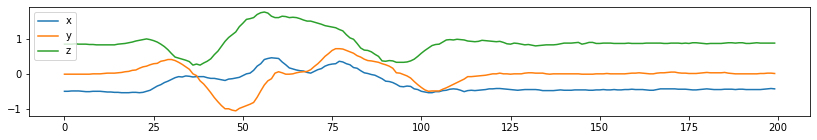Finally, save the raw data in a file:

Copy Code
`# Save raw accelerometer datanp.save(label_name, accel_data)`

Just as a test, we can read and print out the raw readings:

Copy Code
`# Test: load arraytest_data = np.load(label_name + '.npy')print(test_data)`

The full code for this example can be found here: https://gist.github.com/ShawnHymel/4d2ed5b5e8f6b516d1c5f7e52d40bf68#file-read_msa301_arduino-ipynb

Binho Nova DAQ with Python

The Binho Nova is a host adapter, which is a useful device for communicating with various sensors and analog/digital devices from your computer. Normally, host adapters are used to debug problems with devices that rely on digital communications, like SPI and I2C. They are also useful for configuring chips, such as flash or EEPROM, prior to assembly during manufacturing.

That being said, they can also be used as an alternative to a DAQ device. The Binho Nova is an inexpensive host adapter that works with CircuitPython. As a result, we can use many of the Adafruit (and community) CircuitPython libraries with the Binho Nova to communicate with various sensors.

Please note that at this time, I2C writes with CircuitPython seem to be somewhat slow with the Binho Nova. I found that it took about 40 ms between I2C reads/writes. Raw communication with the Binho Nova is much faster, if you don’t mind writing your own driver (using raw reads/writes in Python). Hopefully, this will speed up in the future, as being able to use community libraries with a host adapter makes development much easier.

To begin, you’ll need to install the Blinka and Binho Python libraries found on this tutorial.

If you’re using Jupyter Notebook, you’ll need to set an environment variable prior to launching Jupyter Notebook. For example, if you’re using Anaconda, you’ll want to enter one of the following into the Anaconda prompt.

Windows:

Copy Code
`set BLINKA_NOVA=1`

Mac/Ubuntu (in the command prompt before launching Jupyter Notebook):

Copy Code
`export BLINKA_NOVA=1`

After, you can launch Jupyter Notebook:

Copy Code
`jupyter notebook`

In the first cell, if you haven’t already, install the following libraries:

Copy Code
`!python -m pip install binho-host-adapter!python -m pip install adafruit-blinka!python -m pip install adafruit-circuitpython-msa301`

Next, import the necessary packages:

Copy Code
`import boardimport busioimport adafruit_msa301import numpy as npimport matplotlib.pyplot as pltimport time`

Then, create some settings like last time:

Copy Code
`# Settingslabel_name = 'down_up'num_class_samples = 10    # Number of samples to collect for this label categorytime_per_sample = 2.0     # Secondsaccel_sample_rate = 25    # Times per second (Hz) to read from accelerometernum_accel_samples = int(time_per_sample * accel_sample_rate)`

We can initialize communication with the MSA301 sensor through the Binho Nova with the following:

Copy Code
`# Create library object using Bus I2C porti2c = busio.I2C(board.SCL, board.SDA)msa = adafruit_msa301.MSA301(i2c)`

We’ll want to test communication with the sensor with:

Copy Code
`# Test reading from sensorstart = time.time()print(msa.acceleration)print(time.time() - start)`

Just like in the Arduino example, we’ll want to write a function that reads from the sensor for 2 seconds:

Copy Code
`# Connect to Binho Nova and read samplesdef capture_accel(nsamples):    samples = np.zeros((nsamples, 3))    for i in range(nsamples):                # Use timer to determine when to take measurement next        start = time.time()                # Read X, Y, and Z acceleration over I2C and convert to g-force        accel = msa.acceleration        sample = np.array([axis / 9.80665 for axis in accel])        samples[i] = sample                # Wait before reading again        while (time.time() - start) < (1. / accel_sample_rate):            pass            return samples`

We then create a script that captures 10 (or however many you want) samples:

Copy Code
`# Capture sample setaccel_data = np.zeros((num_class_samples, num_accel_samples, 3))i = 0while i < num_class_samples:    input('Press enter and draw shape with board')    try:                # Get sample from Binho Nova        samples = capture_accel(num_accel_samples)        accel_data[i] = np.array(samples)        print('Sample', i, 'captured with shape:', accel_data[i].shape)        i += 1            except:        print('Error parsing samples. Try again.')        pass`

When you run this cell, follow the prompts to press the 'enter' key and perform the gesture in the air with the sensor.

When that’s done, we can plot a few samples with the following:

Copy Code
`# Plot a fewfor sample_idx in range(5):    fig = plt.figure(figsize=(14,2))    ax = fig.add_subplot(111)    ax.plot(accel_data[sample_idx, :, 0], label='x')    ax.plot(accel_data[sample_idx, :, 1], label='y')    ax.plot(accel_data[sample_idx, :, 2], label='z')    plt.legend(loc='upper left')plt.show()`

You should get 5 plots that look something like the following: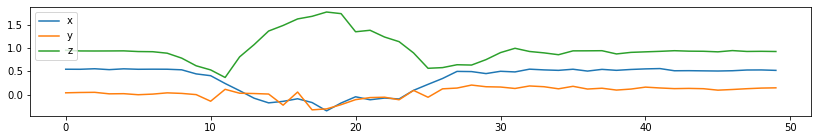Finally, we save the samples as a Numpy array with:

Copy Code
`# Save raw accelerometer datanp.save(label_name, accel_data)`

If we want to test loading the array, we can do that with:

Copy Code
`# Test: load arraytest_data = np.load(label_name + '.npy')print(test_data)`

The full code can be found here: https://gist.github.com/ShawnHymel/8e2e57e76d30939a899b743330df5f10

Going Further

Hopefully, these examples have helped give you some ideas on how you can capture sensor data inexpensively by creating your own DAQ device out of an Arduino or host adapter. While the process might involve a little more code writing than other DAQ options, you can rely on community libraries to simplify the whole process.

The Binho Nova has a number of adapters (e.g. QWIIC) to make interfacing to various sensor boards much easier, some of which can be found here.